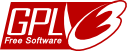# The Sedenion Scientific RPN Calculator

###Software © (2015) John Wayland Bales under the GNU General Public License

Those who are unfamiliar with postfix (reverse Polish) calculation may consult the Wikipedia article.

( a, b )( c, d ) = ( ac - db*, a*d + cb )
e0
e1
e2
e3
e4
e5
e6
e7
e8
e9
e10
e11
e12
e13
e14
e15
Postfix Calculator

Important notice about the numbering of unit basis vectors

The basis vectors satisfy $$e_{2p}=(e_p,0)$$ and $$e_{2p+1}=(0,e_p)$$.

For sedenions $$e_3=e_{0011}\to e_{1100}=e_{12}$$ in traditional numbering.
For octonions (using only $$e_0$$ to $$e_7$$), $$e_3=e_{011}\to e_{110}=e_{6}$$ in traditional numbering.
For quaternions $$e_3=e_{11}\to e_{11}=e_3$$ but $$e_1=e_{01}\to e_{10}=e_2$$.